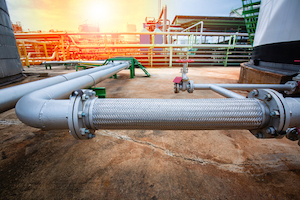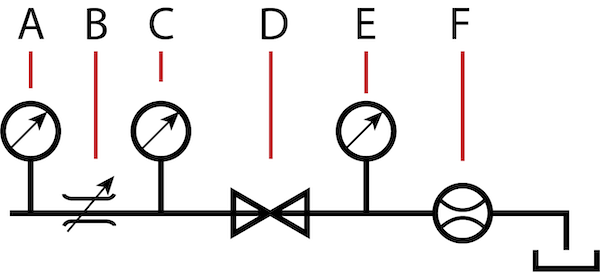# Valve Kv CalculatorFigure 1: Pipes at a oil refinery

The flow coefficient Kv quantifies the fluid flow rate as a function of the pressure differential across the fluid in a system. It is a crucial metric in the sizing of control valves and is expressed in volume per unit of time (e.g., m³/hr or L/min) per unit of pressure drop (e.g., bar or kPa). The higher the Kv value, the greater the fluid flow rate for a given pressure drop. Understanding Kv and Kv valve calculation is essential for engineers who design and maintain fluid control systems, as it helps them to predict the flow rate and select the appropriate valve for a specific application.

## Kv-value calculator fluids & gases

• Fluids: Fill in a value for the inlet pressure, the outlet pressure, and the minimum required flow rate. Click calculate, and the Cv value and Kv value will be calculated. Choose a valve with a Kv-value equal to or higher than the calculated value.
• Gas: Choose a gas type and the inlet temperature. Choose the inlet pressure, the outlet pressure, the required flow rate, and click calculate. Choose a valve with an equal or higher Kv-value to achieve the desired flow rate. The N in the flow rate stands for normal conditions (atmospheric pressure and 0 degrees Celsius).
Liquid:
Specific gravity:
Inlet pressure:
Outlet pressure:
Flow rate:

Valve Kv value:
Valve Cv value:
Gas:
Specific gravity:
Air temperature:
Inlet pressure:
Outlet pressure:
Flow rate:

Valve Kv value:
Valve Cv value:

## What is the flow coefficient Kv

Kv is the flow coefficient in the metric system. It measures the rate at which the fluid (liquid or gas) passes through a valve. It is the volume of water in cubic meters that flows through a valve per hour at a pressure drop of 1 bar with a fully open valve. The Kv value is proportional to the valve's bore size and determines the bore size of a valve required to regulate the flow of a specific fluid. The Kv value is crucial in various applications, such as controlling water flow in heating and cooling systems and ensuring fluid flow through pipes is safe and consistent. A valve flow rate calculator can be used to predict the flow rate of fluid based on the Kv value.

A valve that operates at more positions than open and closed has multiple Kv values. Read our article on Cv calculations to learn more about calculating the flow coefficient in the imperial system.

## Kv calculator

The Kv value determines the correct valve size for a specific application. Once the Kv value is calculated, it is recommended to choose a valve with a Kv value slightly higher than the estimated value. This ensures that the desired flow rate is achieved and provides a safety margin for potential changes in pressure or flow rate. In some cases, the valve coefficient is predetermined, and you can calculate the flow rate suitable for that valve by working the valve flow calculation formula backward.

Kv is expressed differently for liquids and gasses due to their distinct physical properties and behaviors.

• Liquids are nearly incompressible, while gasses are highly compressible, which leads to a different pressure drop across a pipe carrying each of these fluids.
• Liquids have a higher viscosity than gasses, affecting their flow rate.

Hence, the flow rate equations for liquids and gasses are designed to account for these differences and provide a more accurate representation of the flow rate for each fluid type.

### Kv calculator for Liquids

Know the following to use the Kv flow coefficient calculator correctly for liquids:

• the flow rate in l/min or m3/h
• the density of the medium upstream of the valve
• the pressure drop across the valve i.e., the difference between input pressure and back pressure• Q: volume flow in m3/h
• Δp: pressure drop in bar
• ρ: density of the liquid in kg/m3

#### Example

Calculate the Kv of water with a flow rate of 50 m3/h and a pressure differential of 5 bar.

Solution:

• ρ = 1
• Q = 50 m3/h
• Δp = 5 barTherefore, for the application, select a valve with a Kv value equal to or slightly higher than 22.36.

### Kv calculator for Gasses

Kv for gasses is distinguished between a subcritical and supercritical flow condition.

• Subcritical flow: In subcritical flow, the input pressure and the back pressure of the valve determine the flow rate. The greater the back pressure, i.e., the pressure downstream of the valve (p2), the smaller the flow volume.• Supercritical flow: In supercritical flow, the flow rate depends only on the input pressure, thus resulting in a ‘choked’ flow effect. In a supercritical fluid flow, the fluid pressure is greater than the critical pressure, and the fluid velocity is greater than the critical velocity. At this stage, the flow rate becomes independent of the downstream pressure and is only controlled by the upstream pressure and fluid density. This results in a ‘choked’ flow, where the fluid velocity remains constant regardless of any changes in the downstream pressure. In other words, the fluid reaches sonic velocity and cannot be accelerated further, so the downstream pressure does not affect the flow rate. Choked flow occurs in pipelines and other flow systems when the fluid flows through a constricted orifice, such as a nozzle or a restriction in the pipe, and it is a limiting factor in the design of high-pressure fluid systems.Subcritical equationSupercritical equation• p1: input pressure in bar
• p2: back pressure in bar
• Δp: pressure drop in bar
• QN: flow rate, standardized, in m3/h
• ρN: density, standardized, in kg/m3
• T: absolute temperature upstream of the valve (in Kelvin)

#### Example

Calculate the Kv value of oxygen with a flow rate of 1000 m3/h, a pressure drop of 5 bar, an upstream pressure of 60 bar, downstream pressure of 55 bar at 293 kelvin.

• p2 = 55 bar
• p1 = 60 bar
• p2 > (p1)/2; therefore,Substituting the values,Therefore, select a valve with a Kv value equal to or slightly higher than 2.31 for the application.

## Measurement setup for Kv calculationFigure 2: Measurement setup for Kv calculation: temperature-measurement device (A), flow control valve (B), upstream pressure gauge (C), test device (D), backpressure gauge (E), and flow meter (F).

Figure 1 shows an example of determining the Kv values of a valve at different conditions.

• A: Temperature-measurement device. The device measures the temperature for gaseous media.
• B: Flow control valve. The flow control valve can be adjusted to maintain a desired flow rate or volume of fluid flowing through the test device.
• C: Pressure gauge to measure upstream pressure (p1).
• D: Test device. The test device is the valve whose Kv value is to be determined for various flow conditions.
• E: Pressure gauge to measure back pressure (p2).
• F: Flow meter. A flowmeter measures the fluid flow rate and provides the values in real time, which can be used to monitor and control various processes and systems.

## Difference between Cv value & Kv flow coefficients

Cv and Kv are used to measure fluid flow rate through a valve. However, the two have several key differences:

1. Units: Cv is expressed in US gallons per minute (gpm), while Kv is expressed in m³/hr.
2. Pressure drop: Cv is based on one psi (pound per square inch) pressure drop, while Kv is based on a 1 bar pressure drop. This means that Cv and Kv are not directly comparable and must be converted when used in different systems with different pressure drop units.
3. Application: Cv is primarily used in the US and Canada, while Kv is widely used in Europe and other parts of the world.

### Conversion formula for Cv and Kv

It is possible to convert between the flow coefficients Cv and Kv.

Kv = 0.857 * Cv

Cv = 1.165 * Kv

## FAQs

### How to convert Kv to Cv without a calculator?

The value of flow coefficient Cv can be converted to Kv using the relation Kv = 0.857 Cv.

### How to determine control valve Kv in a calculation?

Kv is represented differently for liquids and gasses. Use our Kv flow calculator with precise Kv calculation formulas to determine the Kv value of your valve.